• ## 复数的三角形式与指数形式

万次阅读 多人点赞 2019-10-22 16:46:46
在中学，我们已经学习过复数及其用代数形式a+bi表达的四则运算法则及算律。在《大学数学》中我们学习过建立在实数集合上的微积分——称为实分析；同样，在复数集合上也可以讨论函数、导数、微分、积分等问题，这就是...
在中学，我们已经学习过复数及其用代数形式a+bi表达的四则运算法则及算律。在《大学数学》中我们学习过建立在实数集合上的微积分——称为实分析；同样，在复数集合上也可以讨论函数、导数、微分、积分等问题，这就是大学数学本科（或研究生）专业里一门必修课《复变函数》，因此我们有必要对复数了解得更多些。
1. 复数的三角形式
1.1 复数的幅角与模
我们知道复数a+bi对应着复平面上的点(a, b)，也对应复平面上一个向量（如下图所示）：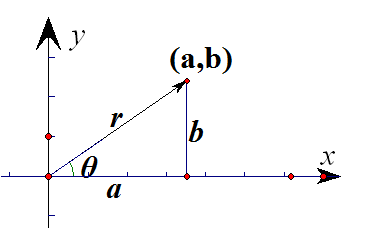这个向量的长度叫做复数a+bi的模，记为|a+bi|，一般情况下，复数的模用字母r表示。同时，这个向量针对x轴的正方向有一个方向角，我们称为幅角，记为arg(a+bi)，幅角一般情形下用希腊字母θ表示。在实轴X与虚轴Y正交的前提下：
式（1）
把它们代入复数的代数形式得：
式（2）
我们把式（2）叫做复数的三角形式。
1.2 复数三角形式的运算法则
引入复数三角形式的一个重要原因在于用三角形式进行乘除法、乘方、开方相对于代数形式较为简单。所以这里只介绍三角形式的乘法、除法、乘方与开方的运算法则。
1.2.1 复数的乘法
设：

则：

这说明，两个复数相乘等于它们的模相乘而幅角相加，这个运算在几何上可以用下面的方法进行：将向量的模扩大为原来的倍，然后再将它绕原点逆时针旋转角，就得到。
1.2.2 复数的除法
设：

则：

这说明，两个复数相除等于它们的模相除而幅角相减，这个运算在几何上可以用下面的方法进行：将向量的模缩小为原来的分之一，然后再将它绕原点顺时针旋转角，就得到。
1.2.3 复数的乘方
利用复数的乘法不难得到：

这说明，复数的n次方等于它模的次方，幅角的倍。这个运算在几何上可以用下面的方法进行：将向量z1的模变为原来的次方，然后再将它绕原点逆时针旋转角，就得到。
1.2.4 复数的开方
对于复数，根据代数基本定理及其推论知，任何一个复数在复数范围内都有n个不同的n次方根。
设：  的一个n次方根为
那么:：
所以：
即：
显然，当k从0依次取到n－1,所得到的角的终边互不相同，但k从n开始取值后，前面的终边又周期性出现。因此，复数z的n个n次方根为:

从求根公式可以看出，相邻两个根之间幅角相差,所以复数的个次方根均匀地分布在以原点为圆心，以它的模的次算术根为半径的圆周上。
因此，求一个复数z的全部n次方根，可以用下面的几何手段进行：

先作出圆心在原点，半径为的圆，然后作出角的终边，以这条终边与圆的交点为分点，将圆周等分，那么每个等分点对应的复数就是复数的次方根。
2. 复数的指数形式
在对复数三角形式的乘法规则讨论中，我们发现，复数的三角形式将复数的乘法“部分地”转变成加法（模相乘，幅角相加），这种改变运算等级的现象在初等函数中有过体现，对数函数与指数函数：

前者将两个同底幂的乘积变成同底的指数相加；后者将两个真数积的对数变成两个同底对数的和。从形式上看，复数的乘法与指数函数的关系更为密切些：

根据这个特点，复数应该可以表示成某种指数形式,即复数应该可以表示成的形式，这里有三个问题需要解决：
（1）反映复数本质特征的三个因素：模r、幅角θ、虚数单位i应各自摆放在什么位置？
（2）在这些位置上它们应呈现什么形态？
（3）作为指数形式的底应该用什么常数？
再重新观察下面的等式：

首先，显然模应该占据中系数的位置，其次，幅角应该占据中指数的位置，对于虚数单位，如果放到系数的位置上会怎样?

等式右边是实数，对于任意虚数而言，这是不可能的。因此幅角θ也应该占据指数的位置。这样第二个问题就产生了：它与幅角一起在指数的位置上是什么关系？（相加？相乘？）
幅角与虚数单位是相加的关系会怎样？先考察模为1的复数，如果写成的形式，一方面由于，与的形式差别不是很大，其次，在复数的乘方法则中，应该仅是幅角的倍而没有虚数单位也要倍，所以虚数单位与幅角不应该是相加关系，而应该是相乘关系。

现在来审查乘法、除法和乘方法则是否吻合：

乘除法保持“模相乘除、幅角相加减”、乘方保持“模的次方、幅角的倍”的本质特征，下面来解决最后一个问题：应该选用哪个常数作为底数？我们暂时将形式化地看做与的“二元函数”，数学是“形式化的科学”，因此，一些形式化的性质应该“形式化”地保持不变。
下面我们将等式两边对形式化地求“偏微分”：

所以，得
这样我们利用不太严格的推理得到了复数的第三种表现形式——指数式

从复数的模与幅角的角度看，复数的指数形式其实是三角形式的简略化，对于指数形式的严格证明可以参读《复数的指数形式的证明》
由复数的三角形式与指数形式，我们很容易得到下面的两个公式：

这两个公式被统称为欧拉公式；在复数的指数形式中，令，，就得到下面的等式：
或者
它是数学里最令人着迷的一个公式，它将数学里最重要的五个数字就这么神秘地联系到了一起：两个超越数——自然对数的底，圆周率；三个单位——虚数单位、自然数的乘法单位和加法单位0。关于自然对数的底和圆周率，这里我想多说那么几句：它们是迄今为止人类所发现的两个彼此独立的超越数，尽管从理论上我们知道，超越数比有理数、代数数（可以表示为有理系数一元多项式的根的数）要多得多，但为人类所认识的超越数却仅此两个！令人不可思议的是，它们居然凭借这么一个简单关系彼此联系着。数学家们评价它是“上帝创造的公式”，我们只能看着它但却不能理解它。
3. 复数的应用
利用复数的三角形式，我们可以比较容易地解决一些数学其他领域里的问题。由于我们这门课的特点，我们仅限于在初等数学领域里举两个例子。
3.1 三角级数求和
求解
解：令，那么对任何自然数有：

另一方面由等比数列的定义可知：

所以：

3.2 设M是单位圆周  x2 +  y2 = 1上的动点，点N与定点A(2,  0)和点M构成一个等边三角形的顶点，并且M→N→A→M成逆时针方向，当M点移动时，求点N的轨迹。
分析：此题若用一般解析几何的方法寻找点M与N之间的显性关系是比较困难的。下面用复数的乘法的几何意义来寻找这种关系。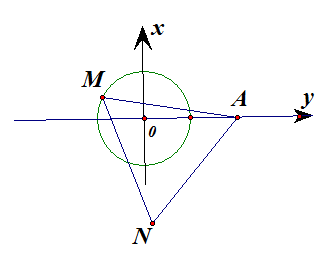设M、N、A对应的复数依次为：，，
那么向量AM可以用向量AN绕A点逆时针旋转300度得到，用复数运算来实现这个变换就是：

3.3 3. 设z1、z2、z3 是复平面上三个点A、B、C对应的复数，证明三角形ABC是等边三角形的充分必要条件是:

假设结论不成立：

三个向量，，均为零向量，则三个向量，，所对应的点是同一个点,与题意不符.
展开全文• 将上面欧拉公式变换成下面复平面的形式： 直角三角形边长公式: 推导出： 正弦、余弦及正切定义式： 上面都是要准备的资料，下面开始推导复数代数形式转换为e指数形式，设有一复数： a + jb ...
根据欧拉公式：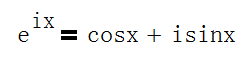公式中，e是自然对数底，i是虚数单位。
将上面欧拉公式变换成下面复平面的形式：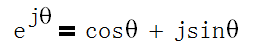直角三角形边长公式: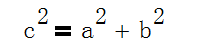推导出：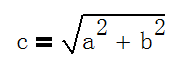正弦、余弦及正切定义式：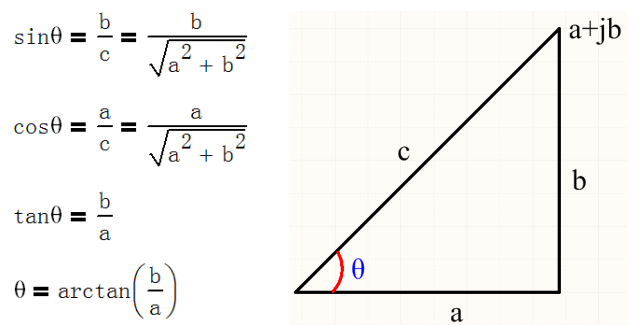上面都是要准备的资料，下面开始推导复数代数形式转换为e指数形式，设有一复数： a + jb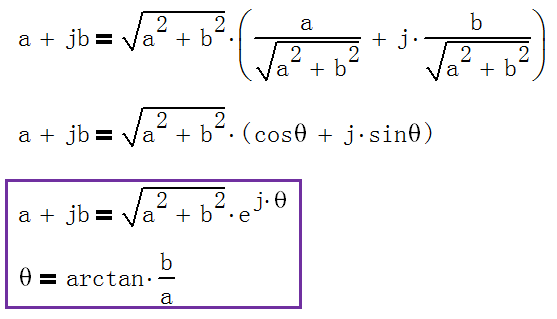展开全文数学
• ## 复数的指数表示

千次阅读 2016-09-08 17:44:56
太丢人了，想当年还学过复变函数呢，时间长了，...z=re^(iθ)； 其中，r为z的模，θ为辐角主值。 z=[(a^2+b^2)^1/2]*{[a/(a^2+b^2)^1/2]+[ib/(a^2+b^2)^1/2]} =r(cosθ+isinθ)=re^(iθ)  (最后一步为欧拉公式)
太丢人了，想当年还学过复变函数呢，时间长了，好多东西都忘了，统统还给了老师！

z=a+ib；
z=re^(iθ)；
其中，r为z的模，θ为辐角主值。
z=[(a^2+b^2)^1/2]*{[a/(a^2+b^2)^1/2]+[ib/(a^2+b^2)^1/2]}
=r(cosθ+isinθ)=re^(iθ)
(最后一步为欧拉公式) 
展开全文• 复数怎么转化为指数形式复数化为三角表示式和指数表示式 复数怎么转化为指数形式相关问题:匿名网友:将复数化为三角表示式和指数表示式是：复数z=a+bi有三角表示式z=rcosθ+irsinθ，可以化为指数表示式z=r*exp(iθ...

导航：网站首页 >
将复数化为三角表示式和指数表示式 复数怎么转化为指数形式
将复数化为三角表示式和指数表示式 复数怎么转化为指数形式
相关问题:
匿名网友:
将复数化为三角表示式和指数表示式是：复数z=a+bi有三角表示式z=rcosθ+irsinθ，可以化为指数表示式z=r*exp(iθ)。exp()为自然对数的底e的指数函数。即：exp(iθ)=cosθ+isinθ。 证明可以通过幂级数展开或对函数两端积分得到，是复变函数的基本公式。
一、三角函数课程介绍：三角函数是以角度为自变量，角度对应任意角终边与单位圆交点坐标或其比值为因变量的函数。也可以等价地用与单位圆有关的各种线段的长度来定义。常见的三角函数包括正弦函数、余弦函数和正切函数。在航海学、测绘学、工程学等其他学科中，还会用到如余切函数、正割函数、余割函数、正矢函数、余矢函数、半正矢函数、半余矢函数等。三角函数一般用于计算三角形中未知长度的边和未知的角度，在导航、工程学以及物理学方面都有广泛的用途。
二、三角函数相关公式：
1、两角和公式
sin(A+B) = sinAcosB+cosAsinB
sin(A-B) = sinAcosB-cosAsinB
cos(A+B) = cosAcosB-sinAsinB
cos(A-B) = cosAcosB+sinAsinB
tan(A+B) = (tanA+tanB)/(1-tanAtanB)
tan(A-B) = (tanA-tanB)/(1+tanAtanB)
cot(A+B) = (cotAcotB-1)/(cotB+cotA)
cot(A-B) = (cotAcotB+1)/(cotB-cotA)
2、倍角公式
tan2A = 2tanA/(1-tan² A)
Sin2A=2SinA•CosA
Cos2A = Cos^2 A--Sin² A
=2Cos² A—1
=1—2sin^2 A
3、三倍角公式
sin3A = 3sinA-4(sinA)³;
cos3A = 4(cosA)³ -3cosA
tan3a = tan a • tan(π/3+a)• tan(π/3-a)
4、半角公式
sin(A/2) = √{(1--cosA)/2}
cos(A/2) = √{(1+cosA)/2}
tan(A/2) = √{(1--cosA)/(1+cosA)}
cot(A/2) = √{(1+cosA)/(1-cosA)} ?
tan(A/2) = (1--cosA)/sinA=sinA/(1+cosA)
5、和差化积
sin(a)+sin(b) = 2sin[(a+b)/2]cos[(a-b)/2]
sin(a)-sin(b) = 2cos[(a+b)/2]sin[(a-b)/2]
cos(a)+cos(b) = 2cos[(a+b)/2]cos[(a-b)/2]
cos(a)-cos(b) = -2sin[(a+b)/2]sin[(a-b)/2]
tanA+tanB=sin(A+B)/cosAcosB
6、积化和差
sin(a)sin(b) = -1/2*[cos(a+b)-cos(a-b)]
cos(a)cos(b) = 1/2*[cos(a+b)+cos(a-b)]
sin(a)cos(b) = 1/2*[sin(a+b)+sin(a-b)]
cos(a)sin(b) = 1/2*[sin(a+b)-sin(a-b)]
7、诱导公式
sin(-a) = -sin(a)
cos(-a) = cos(a)
sin(π/2-a) = cos(a)
cos(π/2-a) = sin(a)
sin(π/2+a) = cos(a)
cos(π/2+a) = -sin(a)
sin(π-a) = sin(a)
cos(π-a) = -cos(a)
sin(π+a) = -sin(a)
cos(π+a) = -cos(a)
tgA=tanA = sinA/cosA
8、万能公式
sin(a) = [2tan(a/2)] / {1+[tan(a/2)]²}
cos(a) = {1-[tan(a/2)]^2} / {1+[tan(a/2)]²}
tan(a) = [2tan(a/2)]/{1-[tan(a/2)]^2}
匿名网友:
1.将复数化为三角表示式和指数表示式
答：将复数化为三角表示式和指数表示式是：复数z=a+bi有三角表示式z=rcosθ+irsinθ，可以化为指数表示式z=r*exp(iθ)。exp()为自然对数的底e的指数函数。即：exp(iθ)=cosθ+isinθ。 证明可以通过幂级数展开或对函数两端积分得到，是复变函数的基本公式...
2.复数怎么转化为指数形式
答：能写成a+bi形式的数叫做复数，其中a和b都是实数，i是虚数单位，i^2=-1。 在复数z=a+bi中，a=Re(z)称为实部，b=Im(z)称为虚部。当虚部等于零时，这个复数可以视为实数；当z的虚部不等于零时，实部等于零时，常称z为纯虚数。 复数有多种表示形式：...
3.复数的指数表示
问：复数的指数形式是怎样的？是什么意思？怎样推出的？
4.复数的指数形式是
问：复数可以写成z=re^iθ的形式，其中虚部和实部是什么，这个公式用语言来讲...
5.指数形式exp到复数形式的转换
问：如下的方程，如何推导得到呢？
6.指数为复数怎么计算啊
问：例如 4^j(-5)我这里的题是 e^j(69度) PS(69度)是角度 这题是交流电...
7.复数的指数形式
问：怎样证明？ 这是什么意思？
8.将下列复数转化为三角表达式和指数表达式。 这两题...
答：解：(4)1-cosφ+isinφ=2[sin(φ/2)]^2+i2sin(φ/2)cos(φ/2)=2sin(φ/2)[sin(φ/2)+icos(φ/2)]=2sin(φ/2)[cos(π/2-φ/2)+isin(π/2-φ/2)]=2sin(φ/2)e^[(π/2-φ/2)i]。 (5)(cos5φ+isin5φ)^2=[e^(i5φ)]^2=e^(i10φ)；(cos3φ-isin3φ)^3=[e^(-i3φ)]^3=e^(-...
9.mathematica有没有把复数写成指数形式的函数
答：先利用模Abs和辐角Arg将复数转换成三角形式，再利用TrigToExp将三角形式转换成指数形式 例如对于1+i,
10.把复数z=1+根号3i分别化成三角形式、指数形式，
答：
问题推荐

展开全文• 文字 符号 代数形式 z=x+iyz=x+iyz=x+iy 点表示 z=(x,y)z=(x,y)z=(x,y) 向量表示 z=op⃗z=\vec{op}z=op ​ 三角形式 z=r(cos⁡θ+isin⁡θ)z=r(\cos\theta+i\sin\theta)z=r(cosθ+isinθ) 指数形式 z=reiθz=re^{i\...
• 1、那是否应该添加定义”i^2=-1”或是“i=√（-1）”,把上述的实集做成一个更大的数集？ 答案是人们认为没有必要！ 2、人们认为正数开方是有意义的，因为开方的结果在现实中有这样的元算与之对应。正如√2，人们...
• ## 傅里叶级数 三角形式 到 复数形式

万次阅读 多人点赞 2014-09-12 11:31:10
通过欧拉公式可以将 三角函数形式的 傅里叶变换 转为 复数形式： 上图的公式看起来不简洁，我们借助一些符号代换让上式看起来简单一些： Cn的求解，我们已经知道an、bn的求解方法为在对应周期上做积分，Cn和an、...傅里叶级数
• 复数及其运算A)复数的表示(1).x=a+bi,其中a称为实部，b称为虚部(2)或写成复指数形式：x=re^(iθ)其中r称为复数的模，又记为 |x| ；θ称为复数的幅度，又记为Arg(x)。且满足r=√(a^2+b^2) ,tanθ=b/a第一种方式适合...
• 复数：z=x+yi 共轭复数： z‾=x−yi\overline{z}=x-yiz=x−yi z=x+yi=z+z‾2+z−z‾2z=x+yi=\frac{z+\overline{z}}{2}+\frac{z-\overline{z}}{2}z=x+yi=2z+z​+2z−z​ z‾=x−yi=z+z‾2−z−z‾2\overline{z}=x-...
• 笛卡尔形式: z = a + b * i 极坐标形式: z = r * (cos() + i * sin()) = √2+2 =arccos(/)=arcsin(/) =∙cos =∙sin 并完成下面两个自定义函数 void inPolarform(double a, double b, double *r, double *phi);...c语言
• 傅里叶级数的复数形式欧拉公式结论 f(t)=a02+∑n=1∞[ancosnwt+bnsinnwt]f(t)=\frac{a_0}{2}+\sum_{n=1}^{\infty}[a_ncosnwt+b_nsinnwt]f(t)=2a0​​+∑n=1∞​[an​cosnwt+bn​sinnwt] a0=2T∫0Tf(t)dta_0=\frac{2}...
• 文章目录指数形式指数形式推导参考 指数形式 根据欧拉公式： eiθ=cos⁡θ+isin⁡θ e^{i\theta } = \cos \theta +i\sin \theta \\ eiθ=cosθ+isinθ 我们可以推出： sin⁡θ=eiθ−e−iθ2icos⁡θ=eiθ+e−iθ2 \...信号处理 深度学习 傅立叶分析
• 下面主要介绍傅里叶级数的理论推导，以及由欧拉方程进一步推导出来的复数形式的傅里叶级数。 1.公式推导用到的结论 函数的周期为T ∫−T/2T/2sin(nx)=0,n=±0,±1,...\int^{T/2}_{-T/2}sin(nx) = 0, n=\pm0,\pm1,......
• ## 复数

千次阅读 2019-04-30 14:14:44
我们把形如z=a+bi（a，b均为实数）的数称为复数，其中a称为实部，b称为虚部，i称为虚数单位。当虚部等于零时，这个复数可以视为实数；当z的虚部不等于零时，实部等于零时，常称z为纯虚数。复数域是实数域的代数闭包...
• 通过下面对比可以发现，用复指数表示复数在几何上更直观点。 复数的运算 1.加法运算 设z1=a+bi，z2=c+di是任意两个复数， 则它们的和是 (a+bi)+(c+di)=(a+c)+(b+d)i。 几何上满足平行四边形法则。 2.乘法运算 设...
• 把小数表示成各个位数及其十进制指数形式（但丢失符号位） FromDigits 从RealDigits的结果，还原这个数，但表示为有理数形式（无法恢复丢失的符号位信息） Sign 考虑到符号的丢失，需要通过Sign函数...函数 double rational conversion
• 复数复数：集合符号为C ，包含实数和虚数。我们把形如z = a + bi(a,b均为实数)的数称为复数。其中a称为实部，b称为虚部，i称为虚数单位。 实数： 实数：集合符号R，由有理数和无理数构成。 虚数： 形如z...
• 在开始傅里叶变换的推导前，我们先使用欧拉公式把傅里叶级数变成指数形式的，因为指数的积分微分更简单。 关于欧拉公式网上有很多推导视频，如果不想看纯数学推导的同学，可以参考这张图： 这个螺旋前进的轨迹，从...傅立叶分析
• 也可以写成极坐标下的指数形式 (R×e​(Pi)​​)，其中 R 是复数模，P 是辐角，i 是虚数单位，其等价于三角形式 R(cos(P)+isin(P))。 现给定两个复数的 R 和 P，要求输出两数乘积的常规形式。 输入格式： 输入在一行...c++ c语言 c#
• 也可以写成极坐标下的指数形式 (R×e​(Pi)​​)，其中 R 是复数模，P 是辐角，i 是虚数单位，其等价于三角形式 (R(cos(P)+isin(P))。 现给定两个复数的 R 和 P，要求输出两数乘积的常规形式。 输入格式： 输入在...
• ## Maxima 中的复数运算

千次阅读 2012-08-02 17:52:03
在 Maxima 中用 %i 表示单位虚数。四则运算和大多数的函数直接就支持复数。比如下面的例子： rectform 函数可以将输入的复数化为标准的...demoivre 函数利用 De Moivre 公式将带复数指数函数化为三角函数形式
• 复数运算 ...在复数a＋bi中，a称为复数的实部，b称为复数的虚部，i称为虚数单位。当虚部等于零时，这个复数就是实数；当虚部不等于零时，这个复数称为虚数，虚数的实部如果等于零，则称为纯虚数。由......